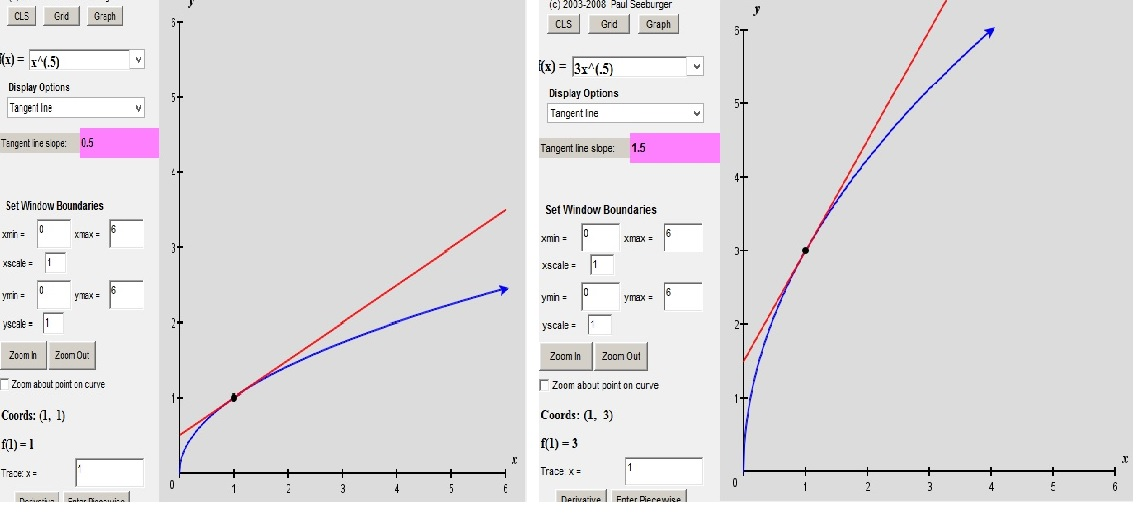# Derivatives, Part II: Combining the Functions

In the last post, we compiled a small collection of derivative formulas, formulas which will enable you to find slopes of tangent lines.  (Why you would want to do such a thing is a discussion that is coming soon.)

You will see all of those functions in various settings as you study AP Physics.  But they don’t always act alone.  You have to be able to work with combinations of those functions in some ways that are intuitive but others that are, well, less intuitive.

1. Adding and Subtracting — Just as you would guess

Suppose f(x) =x3 +x2

and you would like to find the derivative.  You might be thinking:

“Wait — I have a rule for each of those terms, separately.  Can I just add them? ”

In other words, is f'(x) = 3x2 + 2x ?   Yes it is.  We could say this more officially:

If h(x) = f(x) + g(x) then h'(x) = f'(x) + g'(x).

But all that means is that if you have functions added together, you can use their separate derivative formulas and then add them.  And it works the same way for subtraction.

2. Multiplying by a Constant — Also as you would guess

Given f(x) = 3sin(x)…yes, it’s true: f'(x) = 3cos(x).  Multiplying the original function by a constant just multiplies its derivative by the same constant.

There is an intuitive way to see why this must be true:

Consider a function, g(x) = a·f(x), where a>0.   How does the graph of g compare to the graph of f? [SAT fun fact!]  It is vertically stretched or vertically compressed, depending on whether a is greater or less than one.  Now, how would that affect the tangent line?  A vertical stretch by a factor, a, will increase the slope of that tangent line by that same factor, a.

Here is an illustration of what I mean:On the left, you see the graph of f(x) = √x and its tangent line at x = 1.  The slope of that tangent line is .5.

And on the right, you see the graph of f(x) = 3√x and its tangent line, also at x = 1.  The graph has been stretched vertically by a factor of 3.  And sure enough, the tangent line is 3 times as steep, or 1.5.

We’ll call that a “proof” and state the rule officially:  if g(x) = a·f(x) then g'(x) = a·f'(x).

3. Multiplying and Dividing Functions: NOT what you would expect

Well, it’s a bad new/good news kind of thing…

Bad news: you can’t just multiply the derivatives.  In other words, if h(x) = f(x)·g(x), it would be convenient if  h'(x) = f'(x)·g'(x).  But, alas.  Though we don’t know what the rule is, we know enough to see what it isn’t.

All we have to do is let f(x) = x3 and let g(x) = x2.  Then h(x) = f(x)·g(x) = x.

We also already know the derivatives: f'(x) = 3x2 and g'(x) = 2x.  When you multiply them, you get. 3x2·2x = 6x3  But we already know that h'(x) = 5x4.  So the product of the derivatives did not match the derivative of the products.  That’s the bad news. And a similar argument can be constructed to show that quotients don’t work that way either.

So what’s the good news?

1.  There are rules that handle these situations.  They are called the product rule and the quotient rule.  I am sure that your math teacher will be happy to teach them to you.

2.  This is where I pause and remind myself what these posts are for.  I am trying to get you ready for your year of AP Physics.  We may need the product rule and the quotient rule before the year is over, but we won’t need them right away.  By the time we do need them, you will have seen them in math class.

On the other hand, there is one more way to combine functions that we will need almost immediately.  So in the next post, we are going to look at something called “the chain rule”.